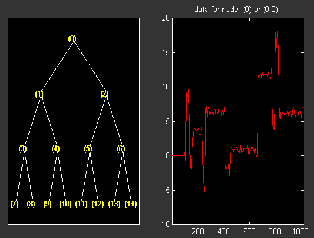## Examples Using Wavelet Packet Tree Objects

You can use command line functions to work with wavelet packet trees (WPTREE objects). The most useful commands are:

We can see some of these features in the following examples.

### plot and wpviewcf

```load noisbump x = noisbump; t = wpdec(x,3,'db2'); fig = plot(t); ```

Click on node 7.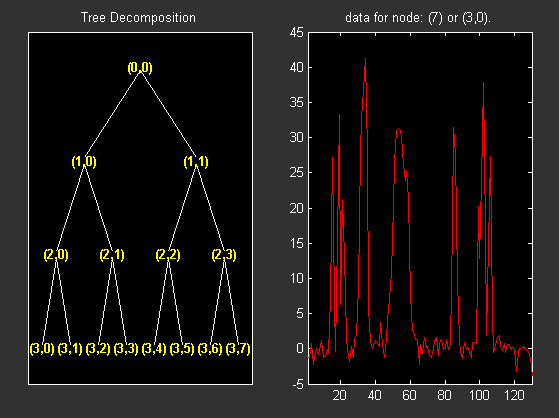Change Node Action from Visualize to Split-Merge and merge the second node.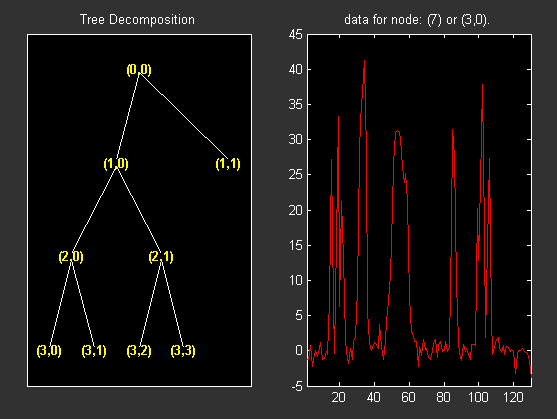```% From the command line, you can get the new tree. newt = plot(t,'read',fig); % The first argument of the plot function in the last command % is dummy. Then the general syntax is: % newt = plot(DUMMY,'read',fig); % where DUMMY is any object parented by an NTREE object. % DUMMY can be any object constructor name, which returns % an object parented by an NTREE object. For example: % newt = plot(ntree,'read',fig); % newt = plot(dtree,'read',fig); % newt = plot(wptree,'read',fig); % From the command line you can modify the new tree, % then plot it. newt = wpjoin(newt,3); fig2 = plot(newt); % Change Node Label from Depth_position to Index and % click the node (3). You get the following figure. ```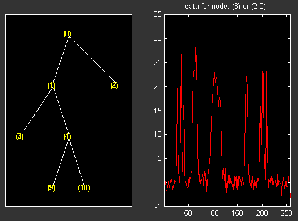```% Using plot(newt,fig), the plot is done in the figure fig, % which already contains a tree object. % You can see the colored wavelet packets coefficients using % from the command line, the wpviewcf function (type help % wpviewcf for more information). wpviewcf(newt,1) % You get the following plot, which contains the terminal nodes % colored coefficients. ```### Change Terminal Node Coefficients

```load gatlin2 t = wpdec2(X,1,'haar'); plot(t); % Change Node Label from Depth_position to Index and % click the node (0). You get the following figure. ```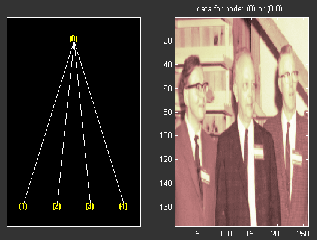```% Now modify the coefficients of the four terminal nodes. newt = t; NBcols = 40; for node = 1:4 cfs = read(t,'data',node); tmp = cfs(1:end,1:NBcols); cfs(1:end,1:NBcols) = cfs(1:end,end-NBcols+1:end); cfs(1:end,end-NBcols+1:end) = tmp; newt = write(newt,'data',node,cfs); end plot(newt) % Change Node Label from Depth_position to Index and % click on the node (0). You get the following figure. ```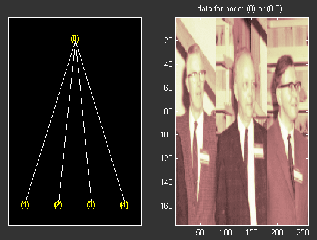You can use this method for a more useful purpose. Let's see a denoising example.

### Thresholding Wavelet Packets

```load noisbloc x = noisbloc; t = wpdec(x,3,'sym4'); plot(t); % Change Node Label from Depth_position to Index and % click the node (0). You get the following plot. ```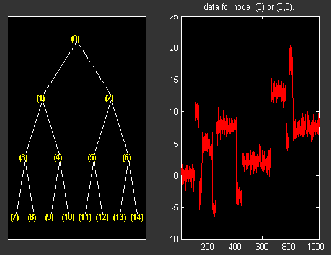```% Global thresholding. t1 = t; sorh = 'h'; thr = wthrmngr('wp1ddenoGBL','penalhi',t); cfs = read(t,'data'); cfs = wthresh(cfs,sorh,thr); t1 = write(t1,'data',cfs); plot(t1) % Change Node Label from Depth_position to Index and % click the node (0). You get the following plot. ```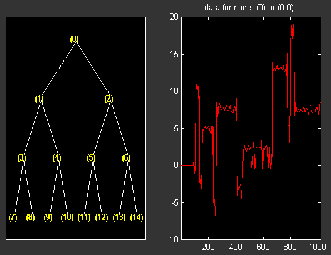```% Node by node thresholding. t2 = t; sorh = 's'; thr(1) = wthrmngr('wp1ddenoGBL','penalhi',t); thr(2) = wthrmngr('wp1ddenoGBL','sqtwologswn',t); tn = leaves(t); for k=1:length(tn) node = tn(k); cfs = read(t,'data',node); numthr = rem(node,2)+1; cfs = wthresh(cfs,sorh,thr(numthr)); t2 = write(t2,'data',node,cfs); end plot(t2) % Change Node Label from Depth_position to Index and % click the node (0). You get the following plot. ```